# ggblanket

## Overview

To simplify ggplot2 visualisation, ggblanket provides:

1. Over thirty gg_* wrapper functions
2. A single col argument to colour and fill by a variable
3. A pal argument to customise colours
4. A facet argument to facet by a variable
5. An additional facet2 argument to facet by a 2nd variable
6. Prefixed arguments to customise scales etc
7. Default titles converted with to sentence case
8. A theme argument to customise the look and feel
9. A gg_theme function to create a quick theme
10. Pretty defaults for symmetry
12. Ability to add geom_* layers
13. Ability to extend further
``````library(dplyr)
library(ggplot2)
library(stringr)
library(ggblanket)
library(palmerpenguins)

penguins <- penguins |>
mutate(sex = str_to_sentence(sex)) |>
tidyr::drop_na(sex)``````

### 1. Over thirty gg_* wrapper functions

Each `gg_*` function wraps a ggplot2 `ggplot(aes(...))` function with the applicable ggplot2 `geom_*()` function. All aesthetics are placed directly in the `gg_*` function: they are not within a `ggplot2::aes` function. This also provides access to the arguments of the relevant stat function.

``````penguins |>
ggplot() +
geom_point(aes(x = flipper_length_mm, y = body_mass_g))``````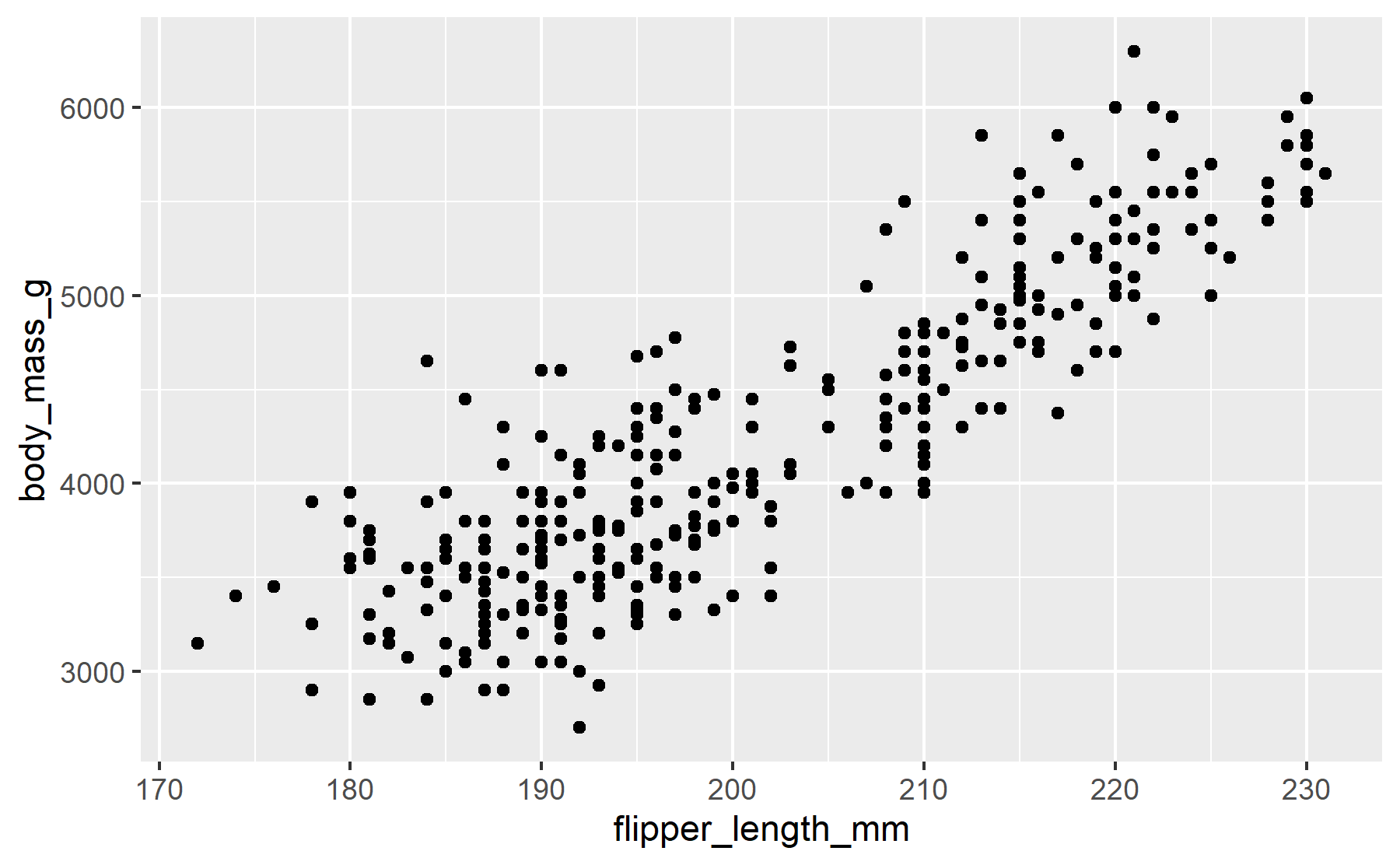``````penguins |>
gg_point(
x = flipper_length_mm,
y = body_mass_g)``````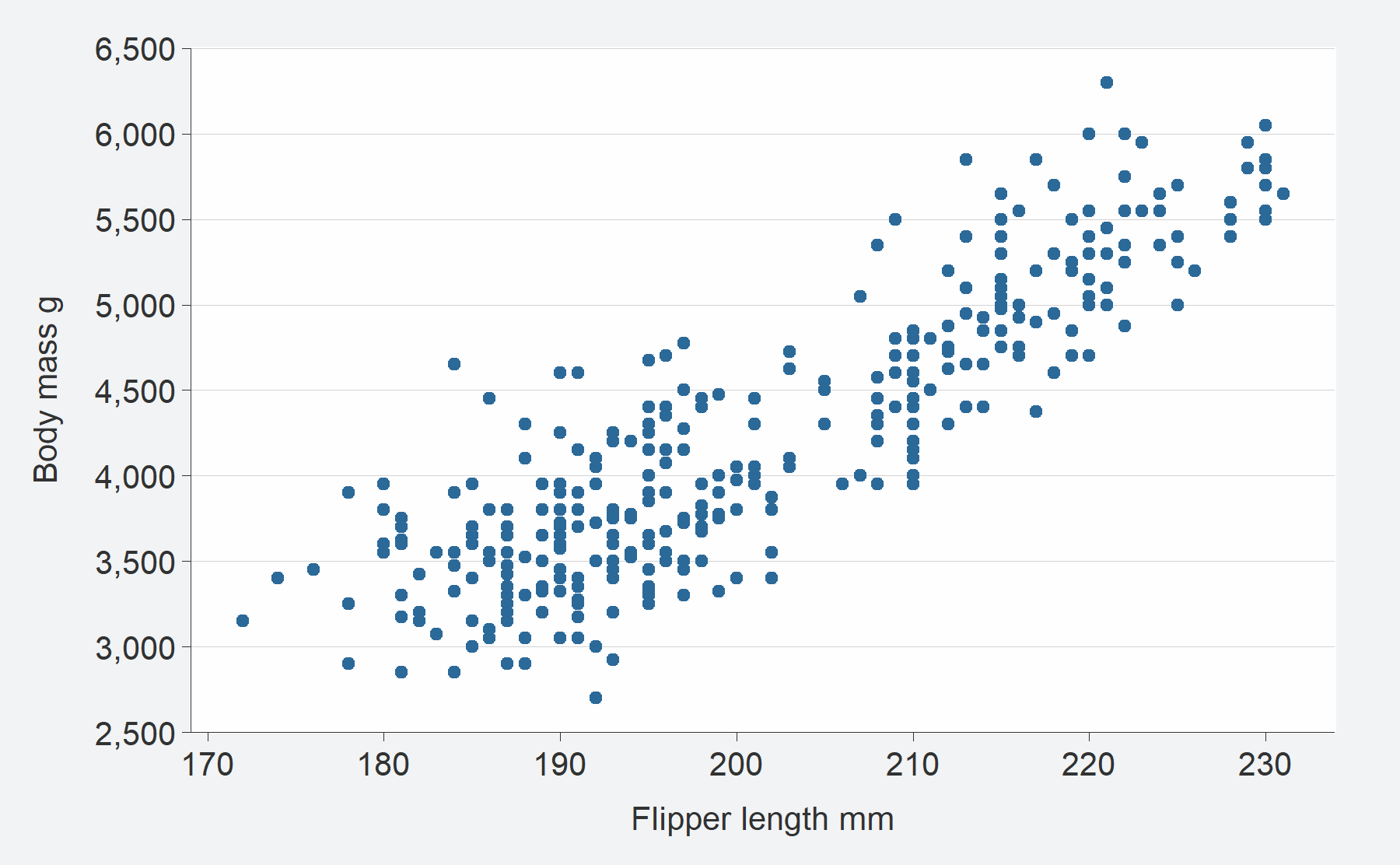### 2. A single col argument to colour and fill by a variable

The colour and fill aesthetics of ggplot2 are merged into a single concept represented by the `col` argument. This always represents the colour of everything in the geom: all points, lines and polygon interiors.

``````penguins |>
ggplot() +
geom_point(aes(x = flipper_length_mm, y = body_mass_g,
col = species))``````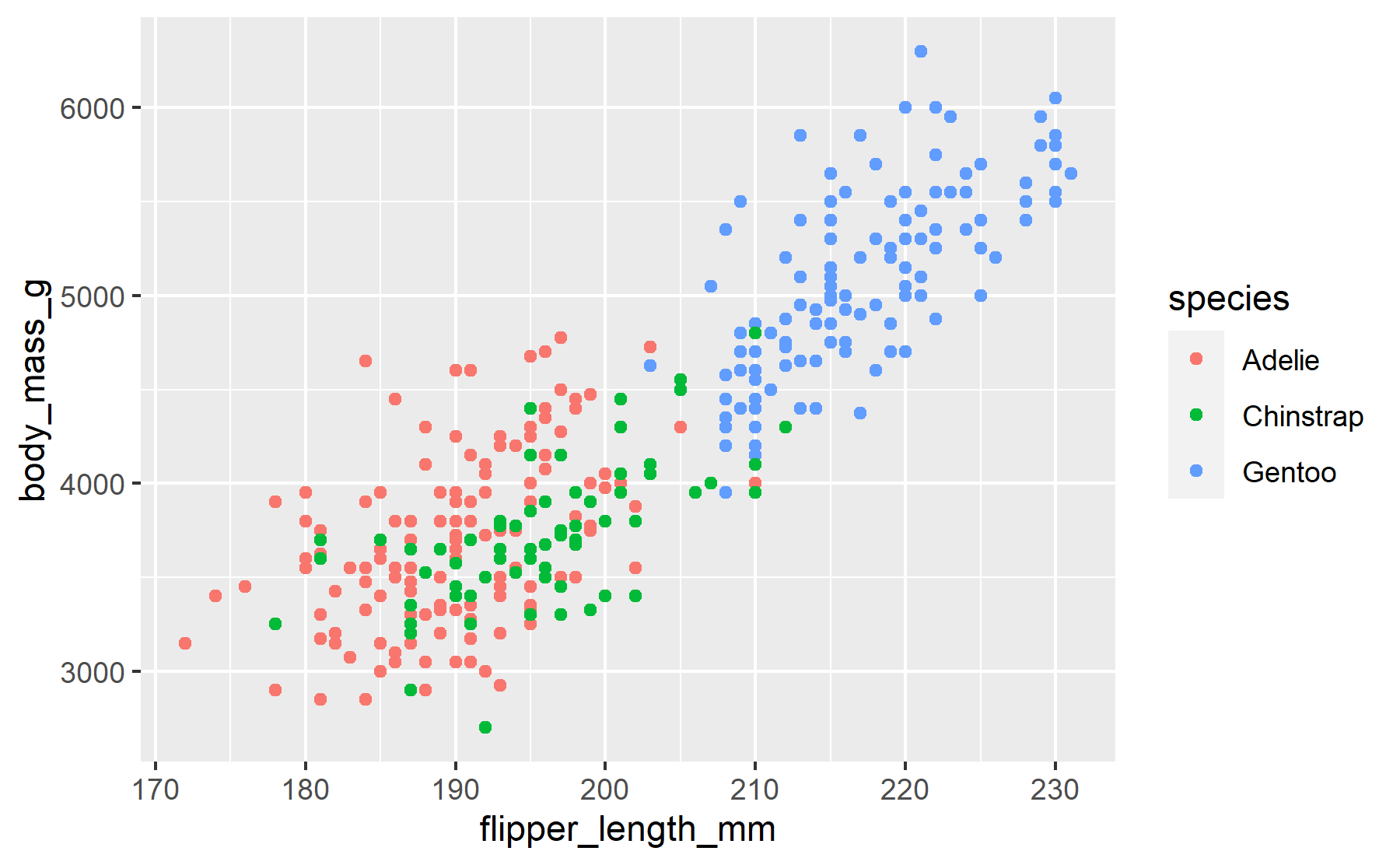``````penguins |>
gg_point(
x = flipper_length_mm,
y = body_mass_g,
col = species)``````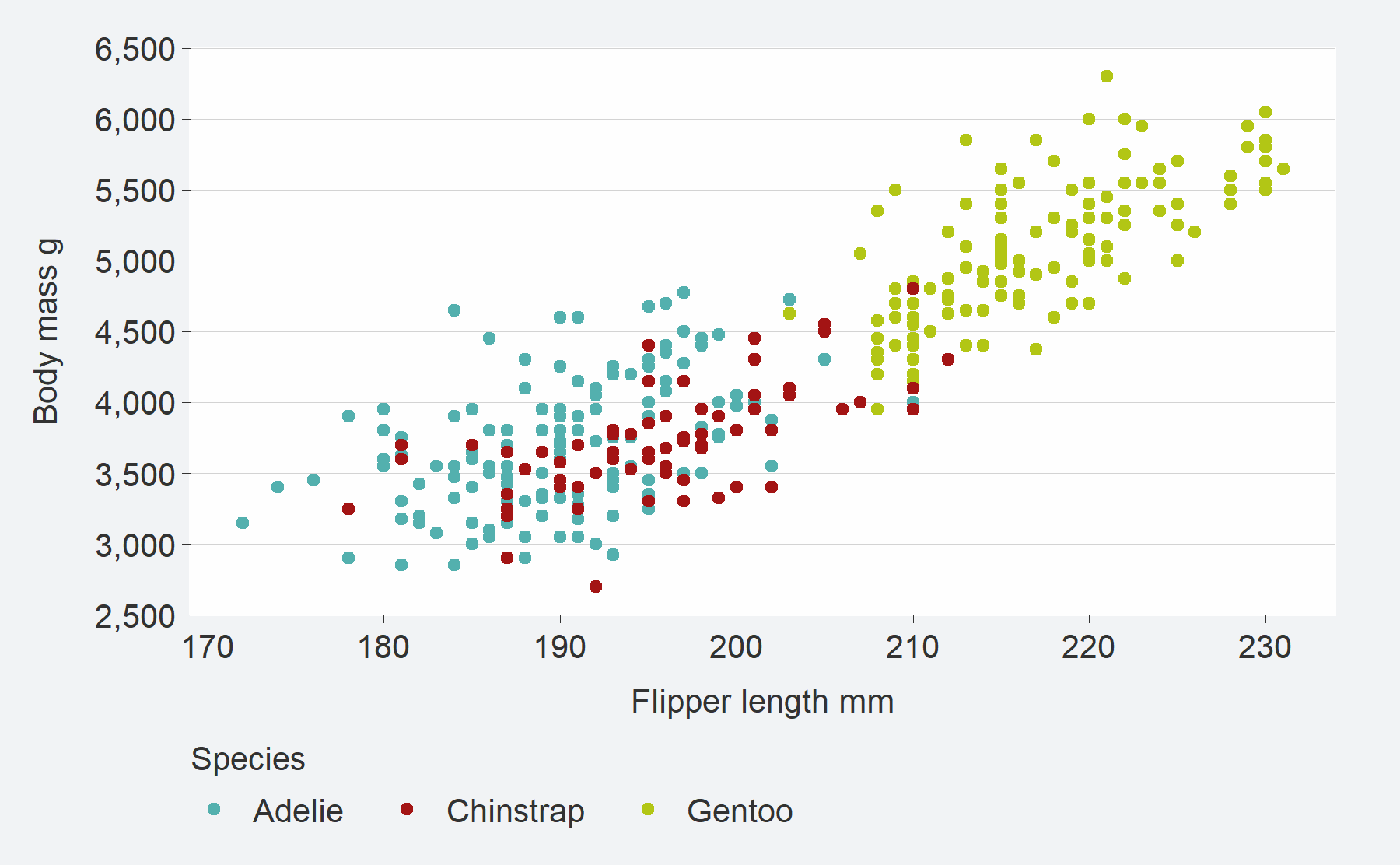``````penguins |>
ggplot() +
geom_density(aes(x = body_mass_g, fill = species))``````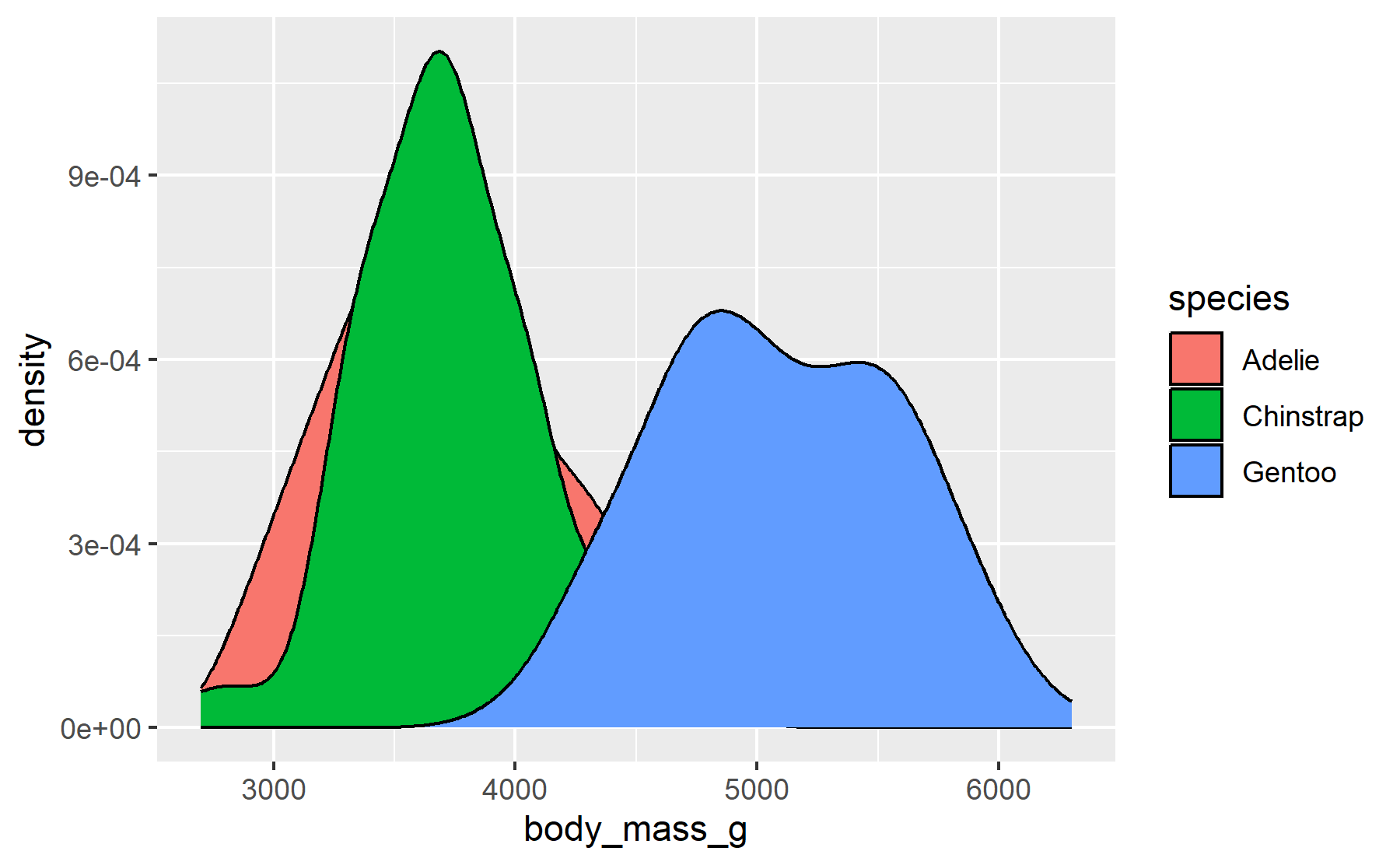``````penguins |>
gg_density(
x = body_mass_g,
col = species)``````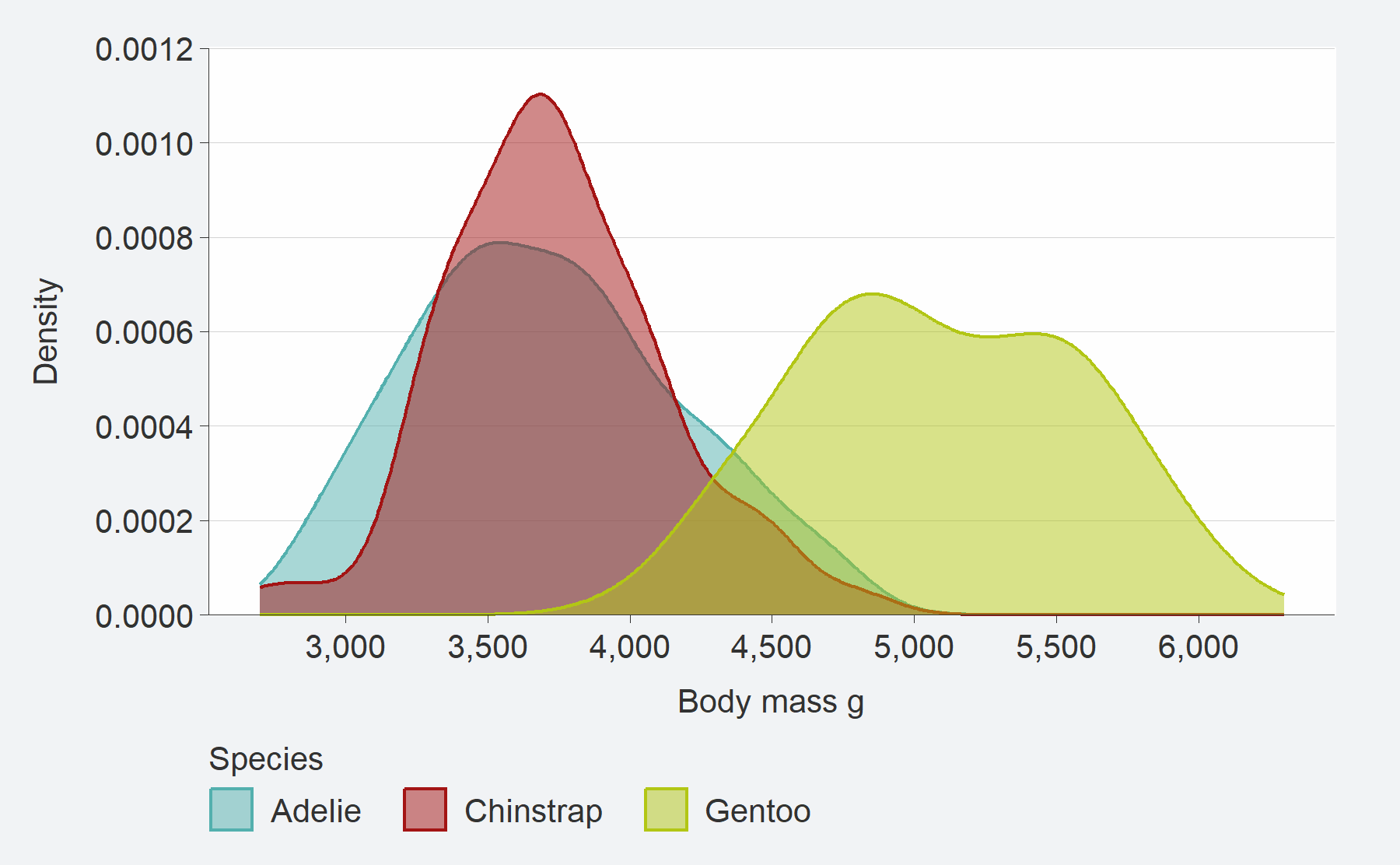### 3. A pal argument to customise colours

The `pal` argument is used to customise the colours of the geom. A user can provide a vector of colours to this argument. It can be named or not. It works in a consistent way - regardless of whether a `col` aesthetic is added or not.

``````penguins |>
ggplot() +
geom_histogram(
aes(x = body_mass_g),
fill = "#1B9E77")``````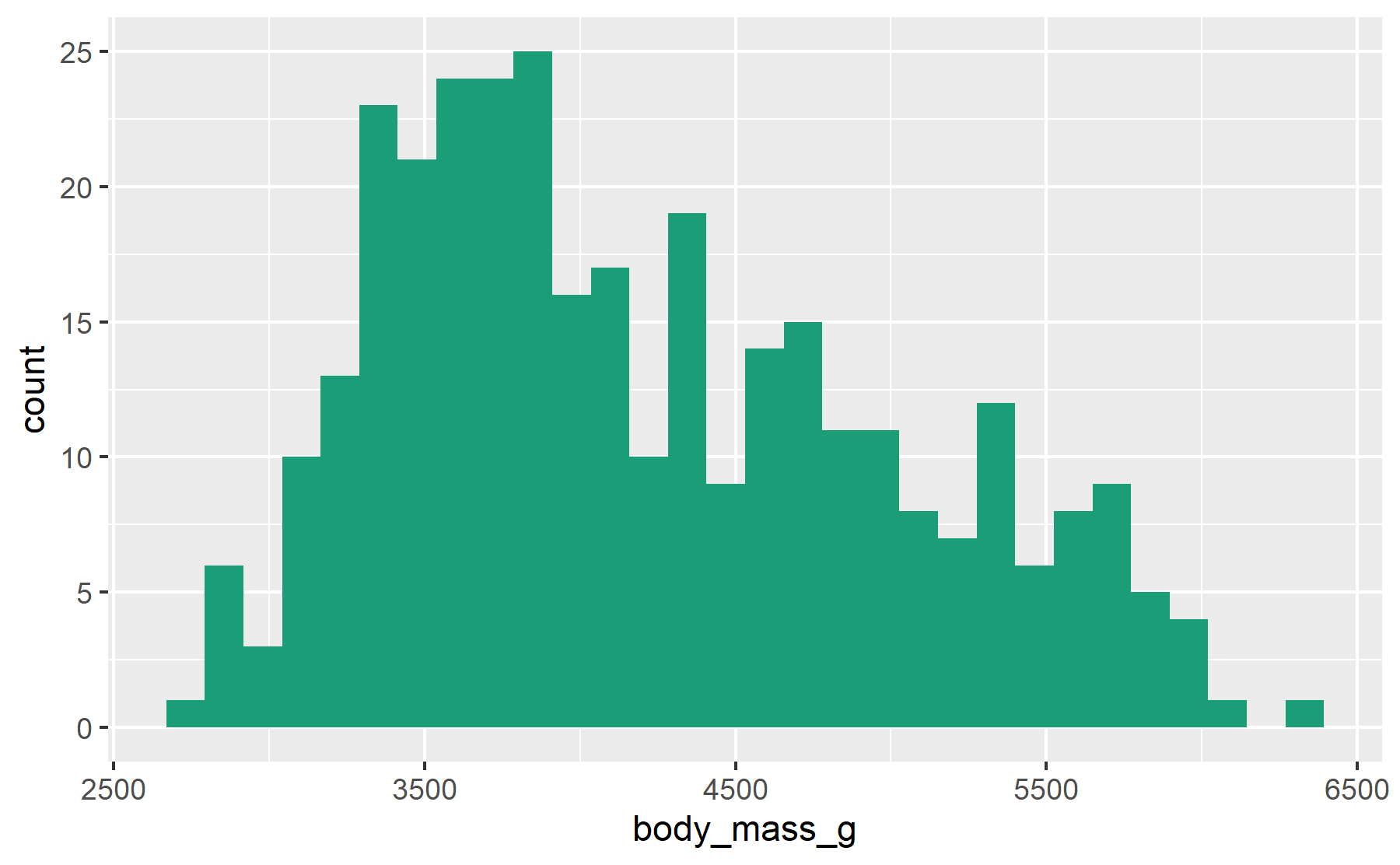``````penguins |>
gg_histogram(
x = body_mass_g,
pal = "#1B9E77")``````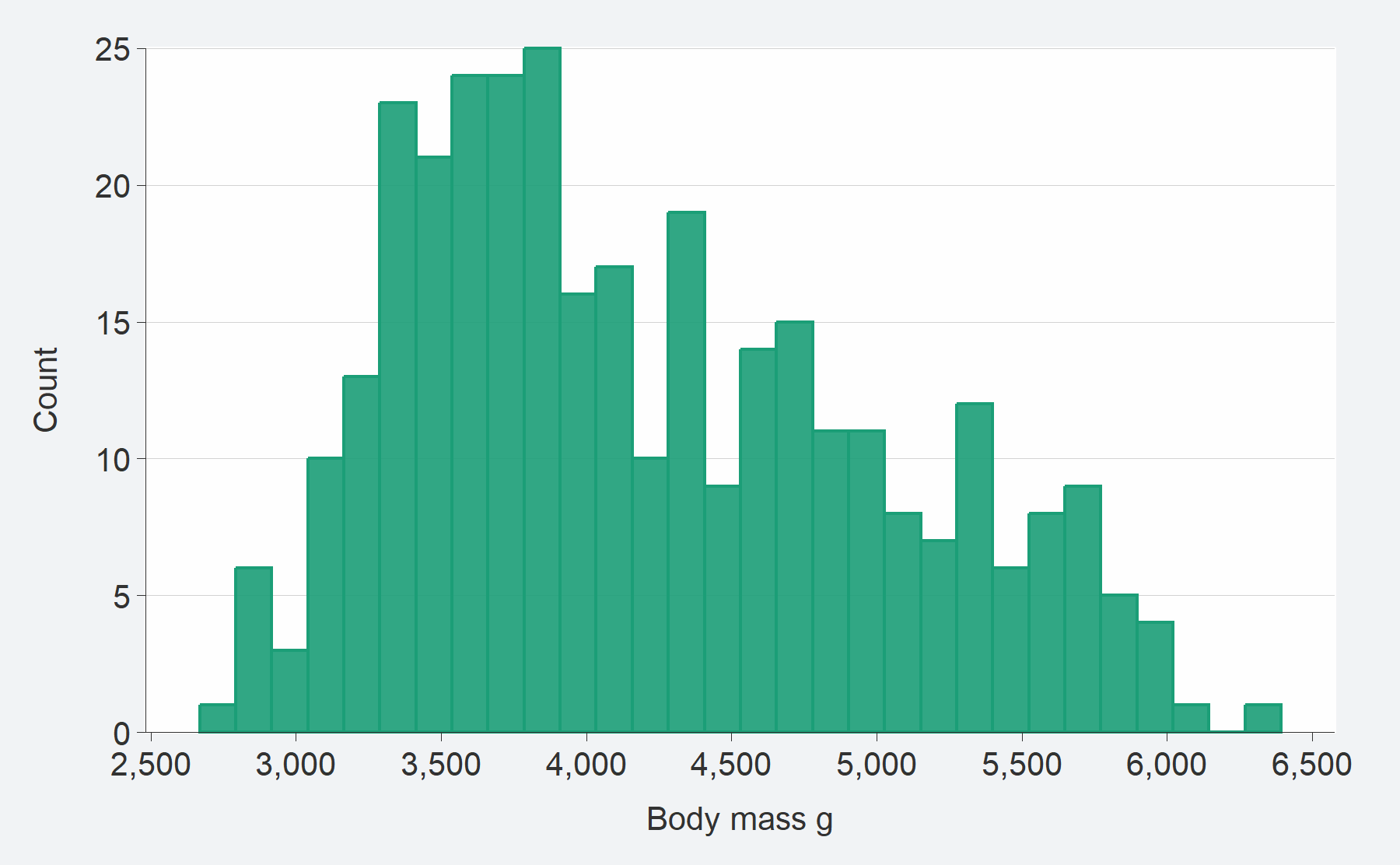``````penguins |>
ggplot() +
geom_jitter(aes(x = species, y = body_mass_g, col = sex)) +
scale_color_manual(values = c("#1B9E77", "#9E361B"))``````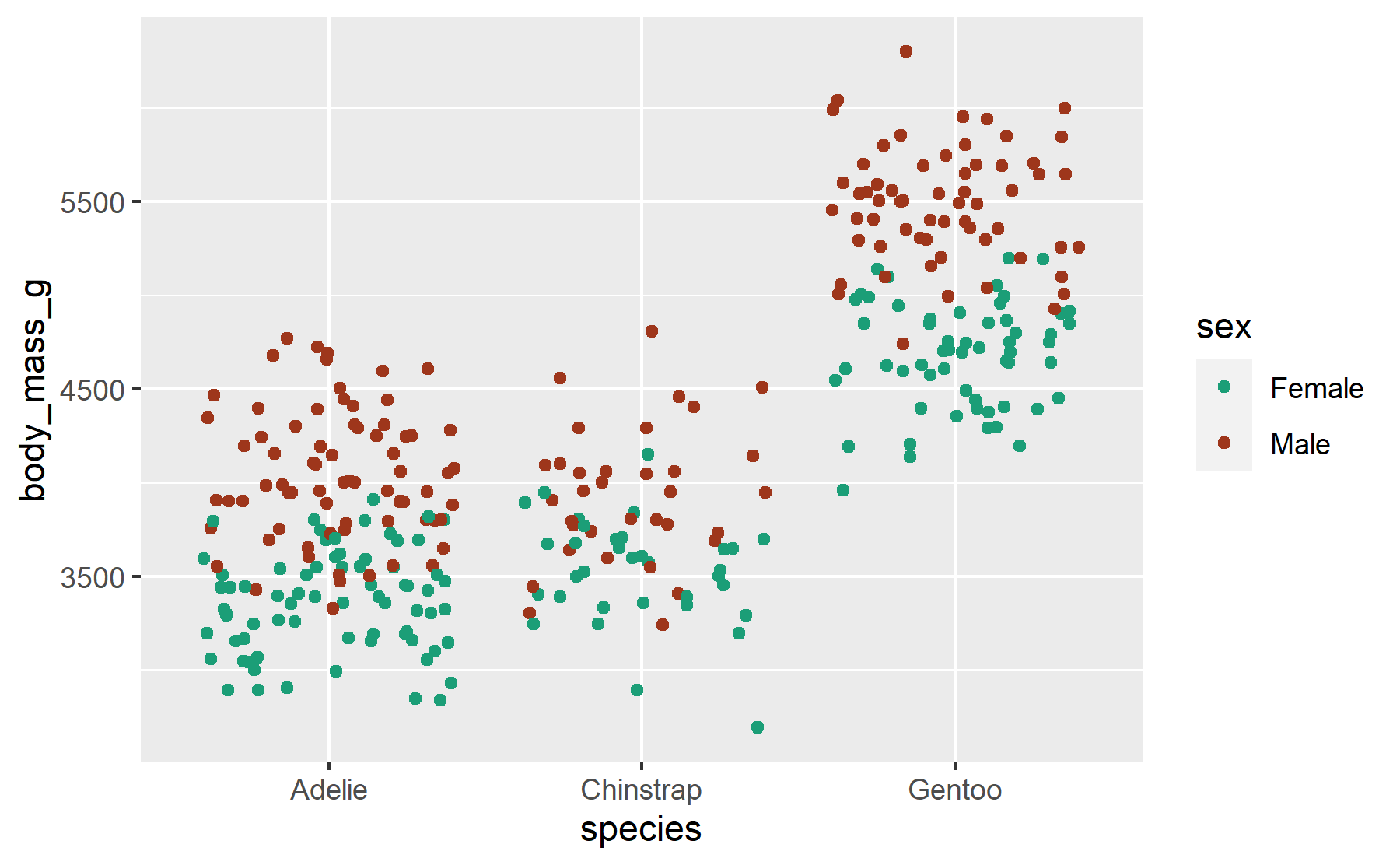``````penguins |>
gg_jitter(
x = species,
y = body_mass_g,
col = sex,
pal = c("#2596be", "#fc7c24"))``````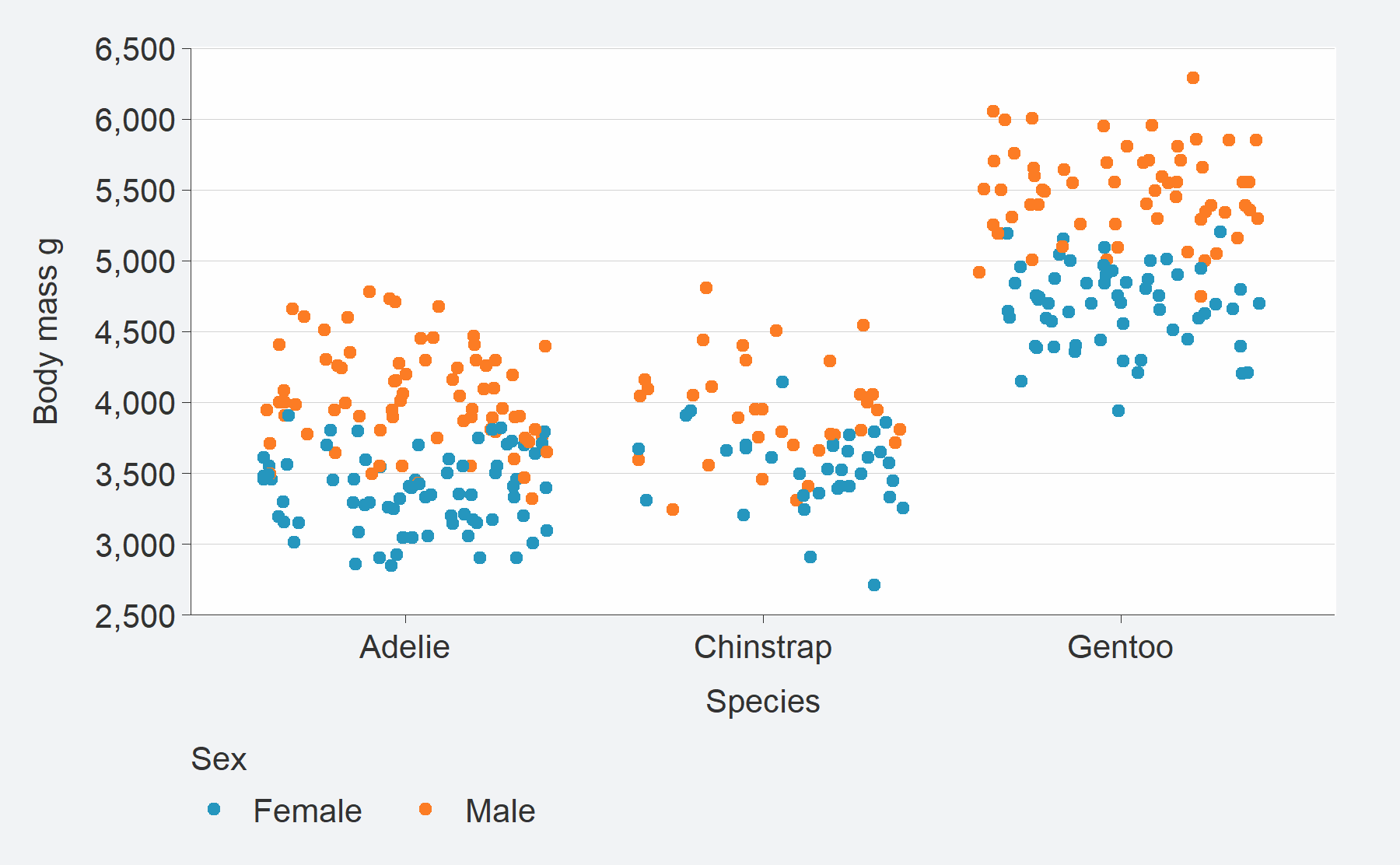### 4. A facet argument to facet by a variable

Faceting is treated as if it were an aesthetic. Users just provide an unquoted variable to facet by. If a single facet (or facet2) variable is provided, it’ll default to a “wrap” layout. But users can change this with a `facet_layout = "grid"` argument.

``````penguins |>
ggplot() +
geom_violin(aes(x = sex, y = body_mass_g)) +
facet_wrap(~species) ``````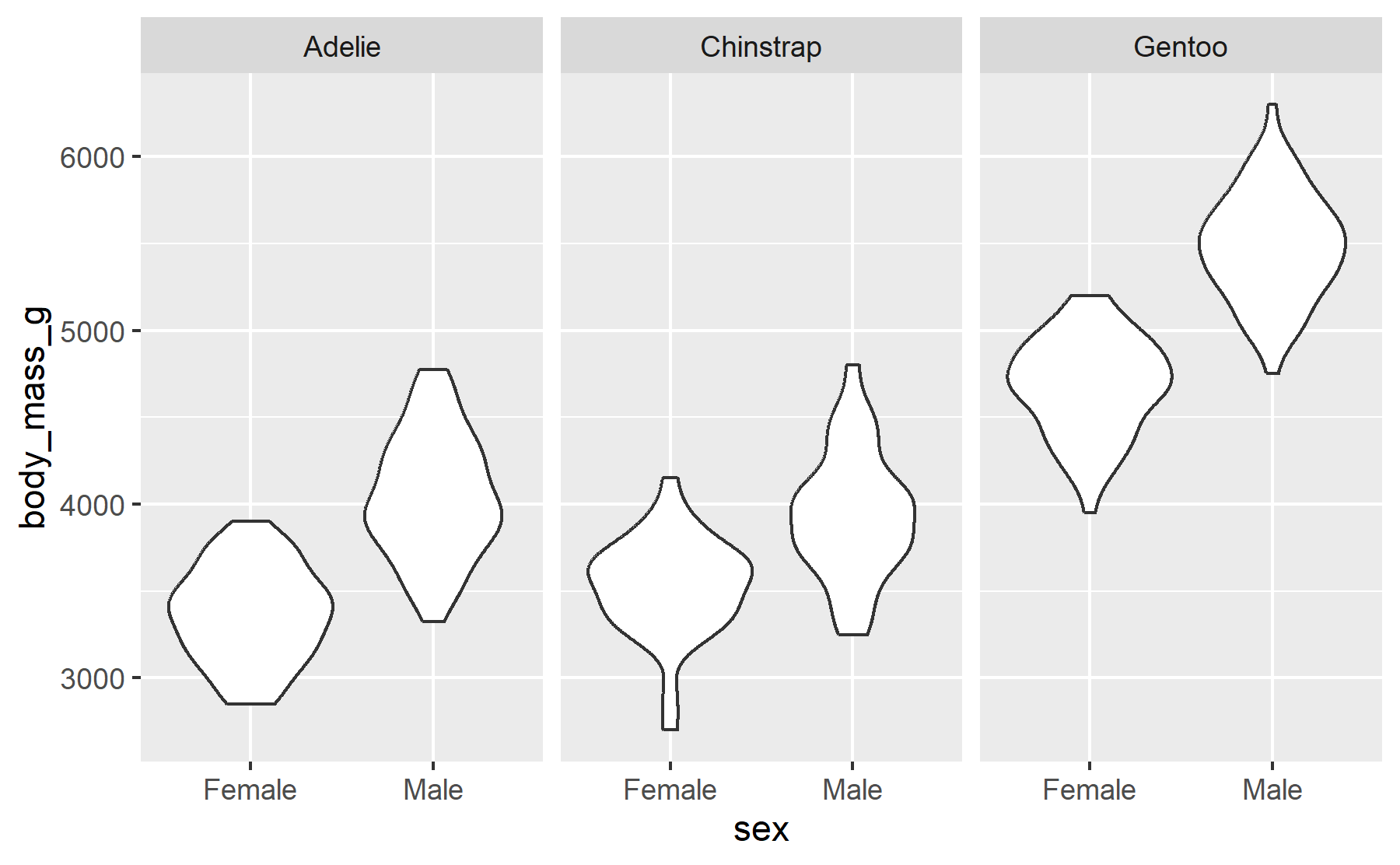``````penguins |>
gg_violin(
x = sex,
y = body_mass_g,
facet = species)``````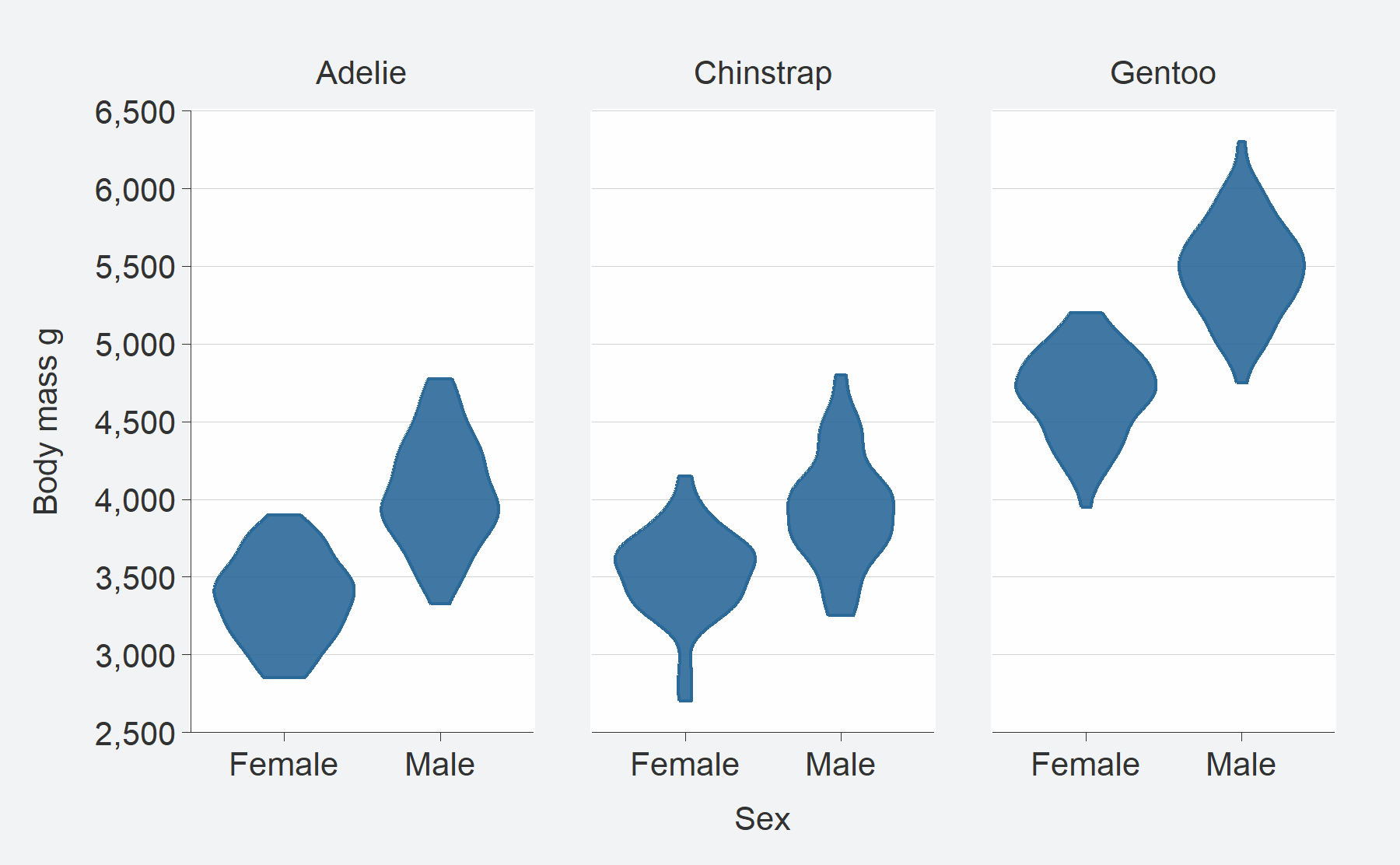### 5. An additional facet2 argument to facet by a 2nd variable

A `facet2` argument is also provided for extra functionality and flexibility. If both `facet`and `facet2` variables are provided, then it’ll default to a “grid” layout of `facet` by `facet2`. But users can change this with a `facet_layout = "wrap"` argument.

``````penguins |>
ggplot() +
geom_histogram(aes(x = flipper_length_mm)) +
facet_grid(species ~ sex)``````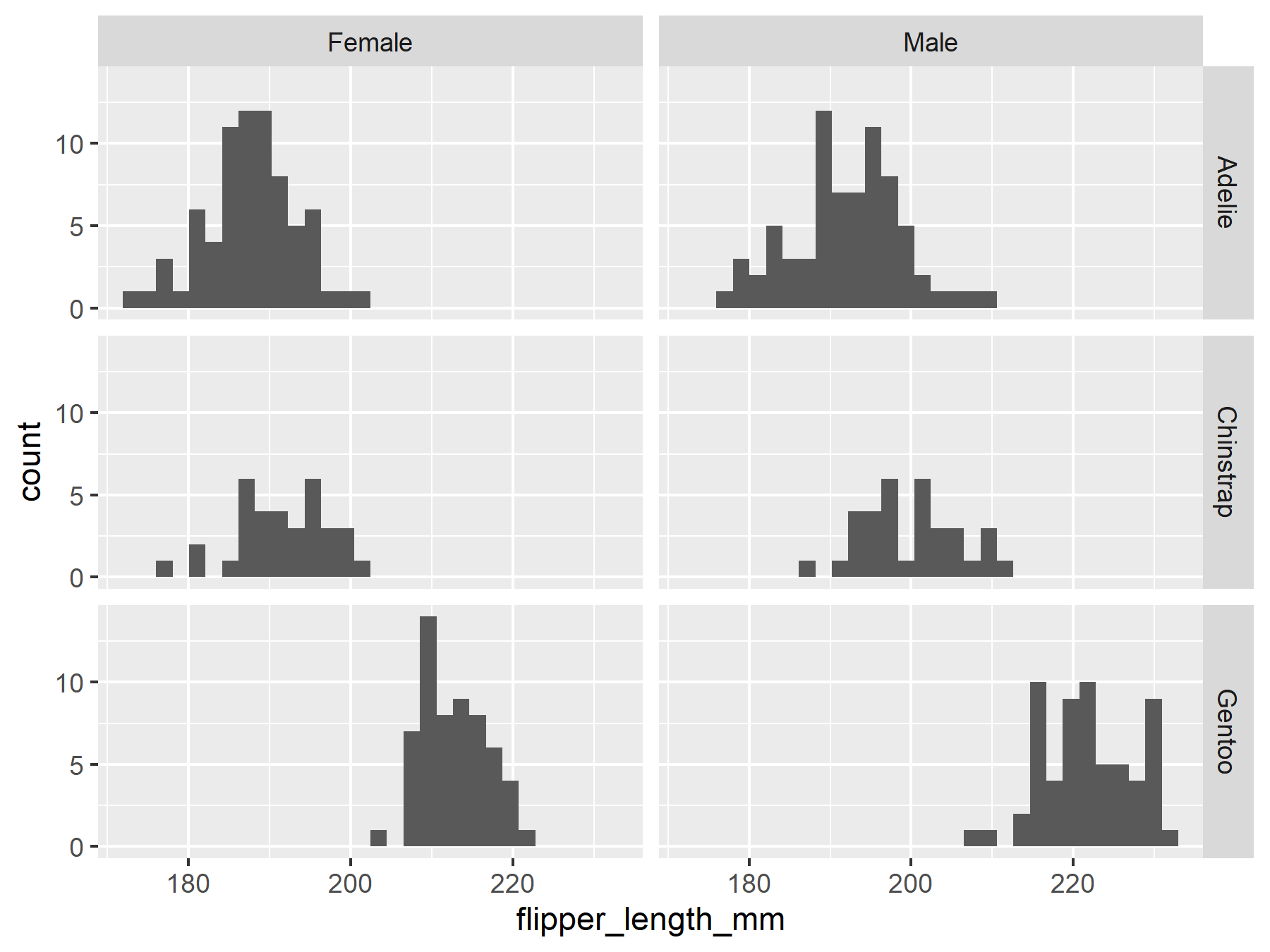``````penguins |>
gg_histogram(
x = flipper_length_mm,
facet = sex,
facet2 = species)``````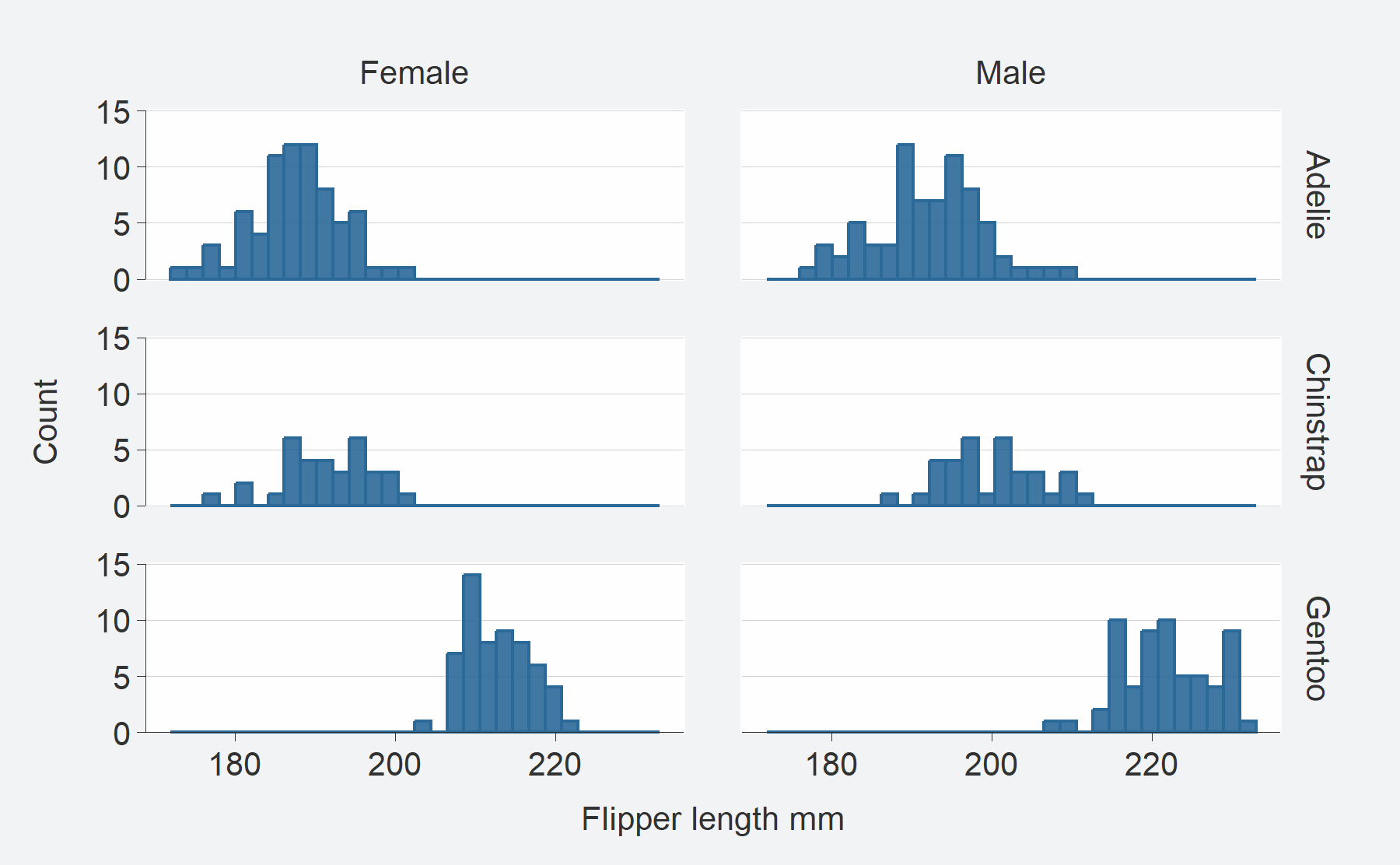### 6. Prefixed arguments to customise scales etc

Prefixed arguments are available to customise scales, guides, titles and faceting etc. These are designed to work with the Rstudio auto-complete to help users remember and find the adjustment they need. Users should first determine whether they want to change something that relates to x, y, col or facet. Then they should type this prefix and press the tab key to access the list of options from the Rstudio auto-complete. Then they can use the arrow keys, and press tab again to select what they want.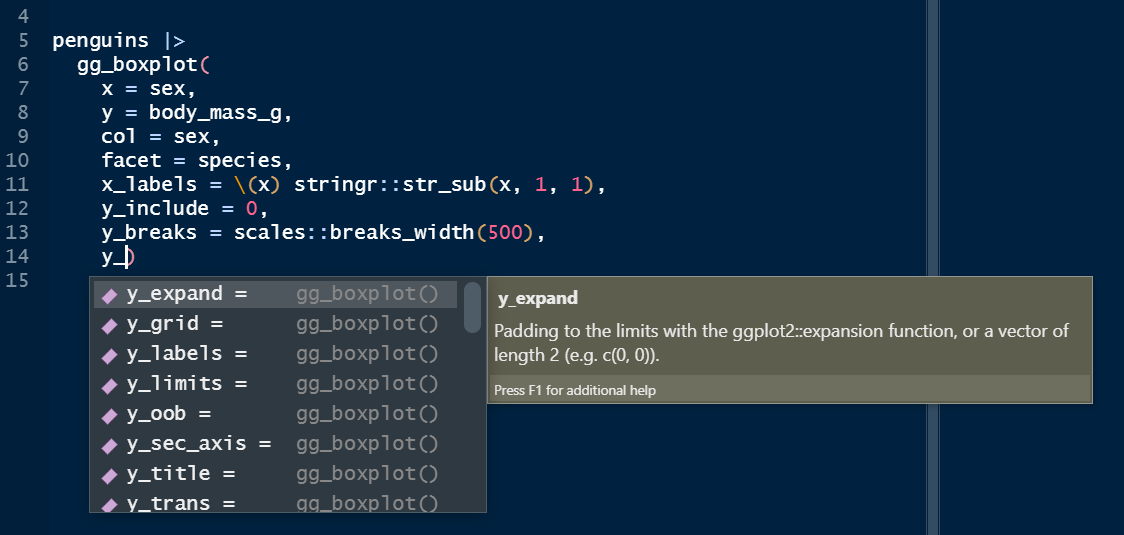``````penguins |>
ggplot() +
geom_jitter(aes(x = species, y = body_mass_g, col = sex)) +
expand_limits(y = 0) +
scale_x_discrete(labels = \(x) str_sub(x, 1, 1)) +
scale_y_continuous(breaks = scales::breaks_width(1500),
labels = scales::label_number(big.mark = " "),
trans = "sqrt") +
labs(x = "Species", y = "Body mass (g)", col = "Sex") +
theme(legend.position = "top") +
theme(legend.justification = "left") +
scale_colour_manual(values = scales::hue_pal()(2),
guide = ggplot2::guide_legend(title.position = "top"))``````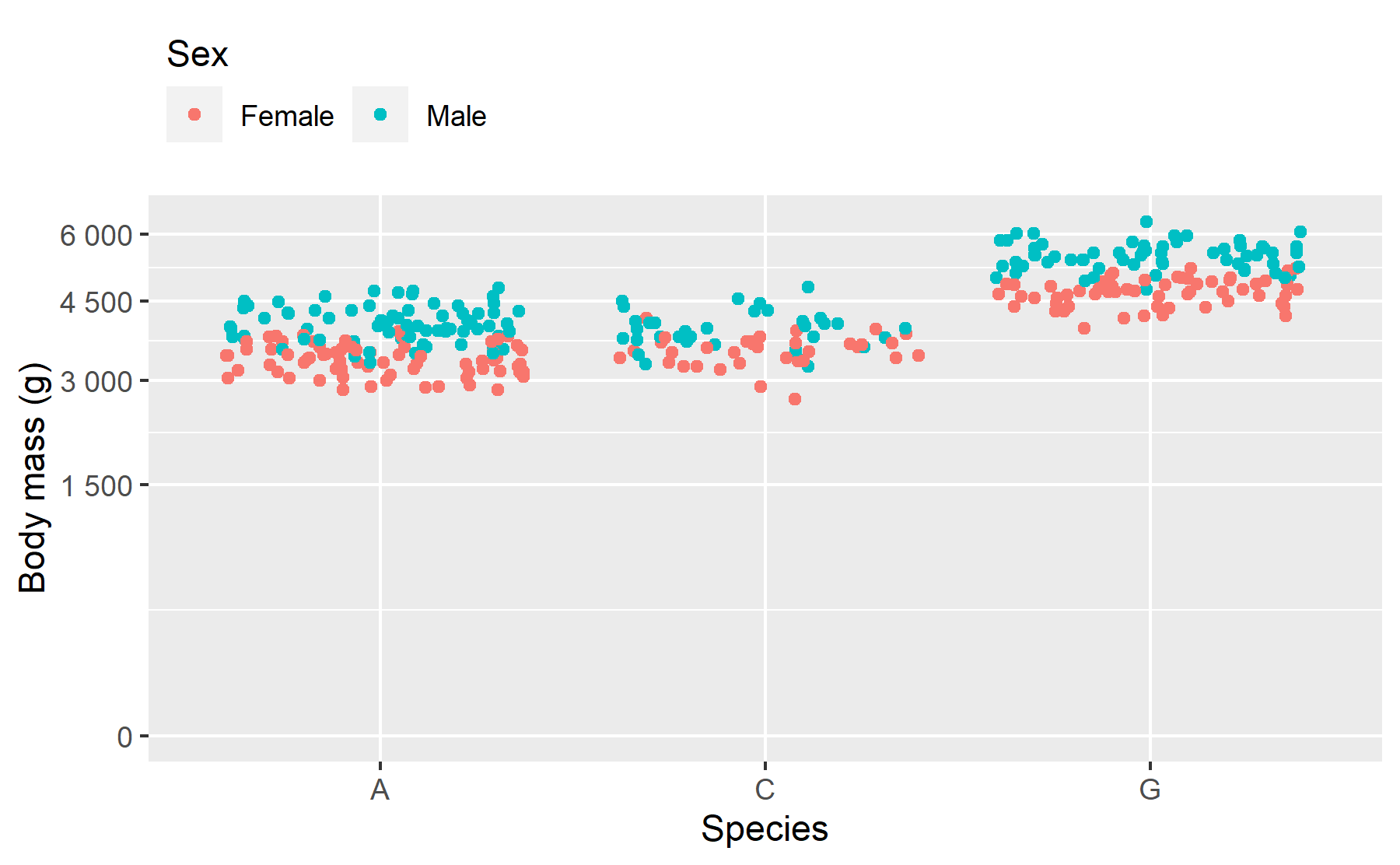``````penguins |>
gg_jitter(
x = species,
y = body_mass_g,
col = sex,
x_labels = \(x) str_sub(x, 1, 1),
y_include = 0,
y_breaks = scales::breaks_width(1500),
y_labels = scales::label_number(big.mark = " "),
y_trans = "sqrt",
y_title = "Body mass (g)",
col_legend_place = "t")``````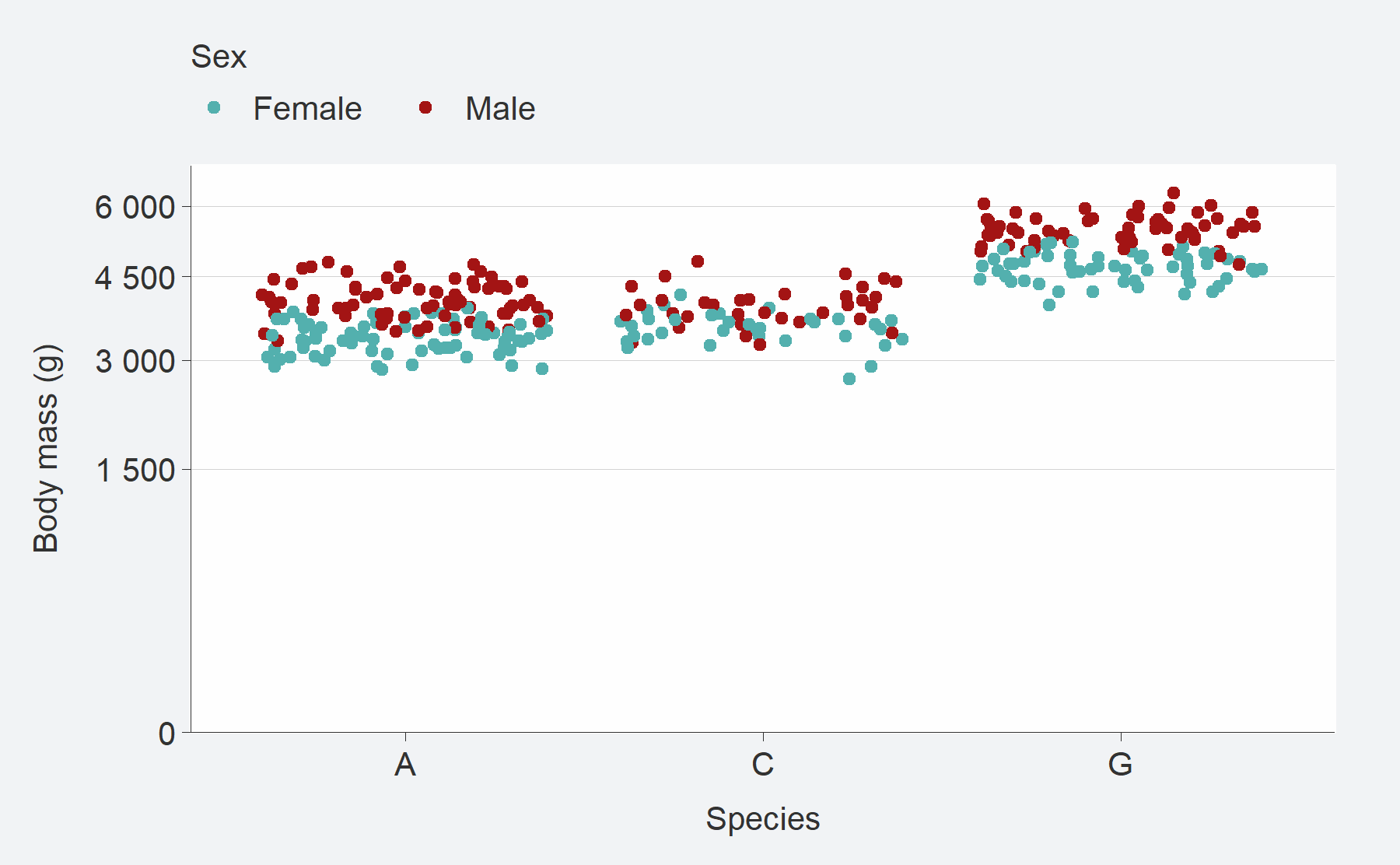``````library(patchwork)
p1 <- penguins |>
gg_point(
x = flipper_length_mm,
y = body_mass_g,
y_include = 0,
x_breaks = scales::breaks_width(25))

p2 <- penguins |>
gg_point(
x = flipper_length_mm,
y = body_mass_g,
y_limits = c(0, NA),
x_breaks = scales::breaks_pretty(3))

p3 <- penguins |>
gg_point(
x = flipper_length_mm,
y = body_mass_g,
x_limits = c(190, 210),
x_oob = scales::oob_keep,
y_trans = "log10",
y_limits = c(1000, NA),
y_breaks = scales::breaks_width(1000),
coord = coord_cartesian(clip = "on"))

p4 <- penguins |>
gg_point(
x = flipper_length_mm,
y = body_mass_g,
x_trans = "reverse",
x_limits = c(210, 190),
x_breaks = scales::breaks_width(-10),
y_include = 0,
y_trans = "sqrt")

(p1 + p2) / (p3 + p4)``````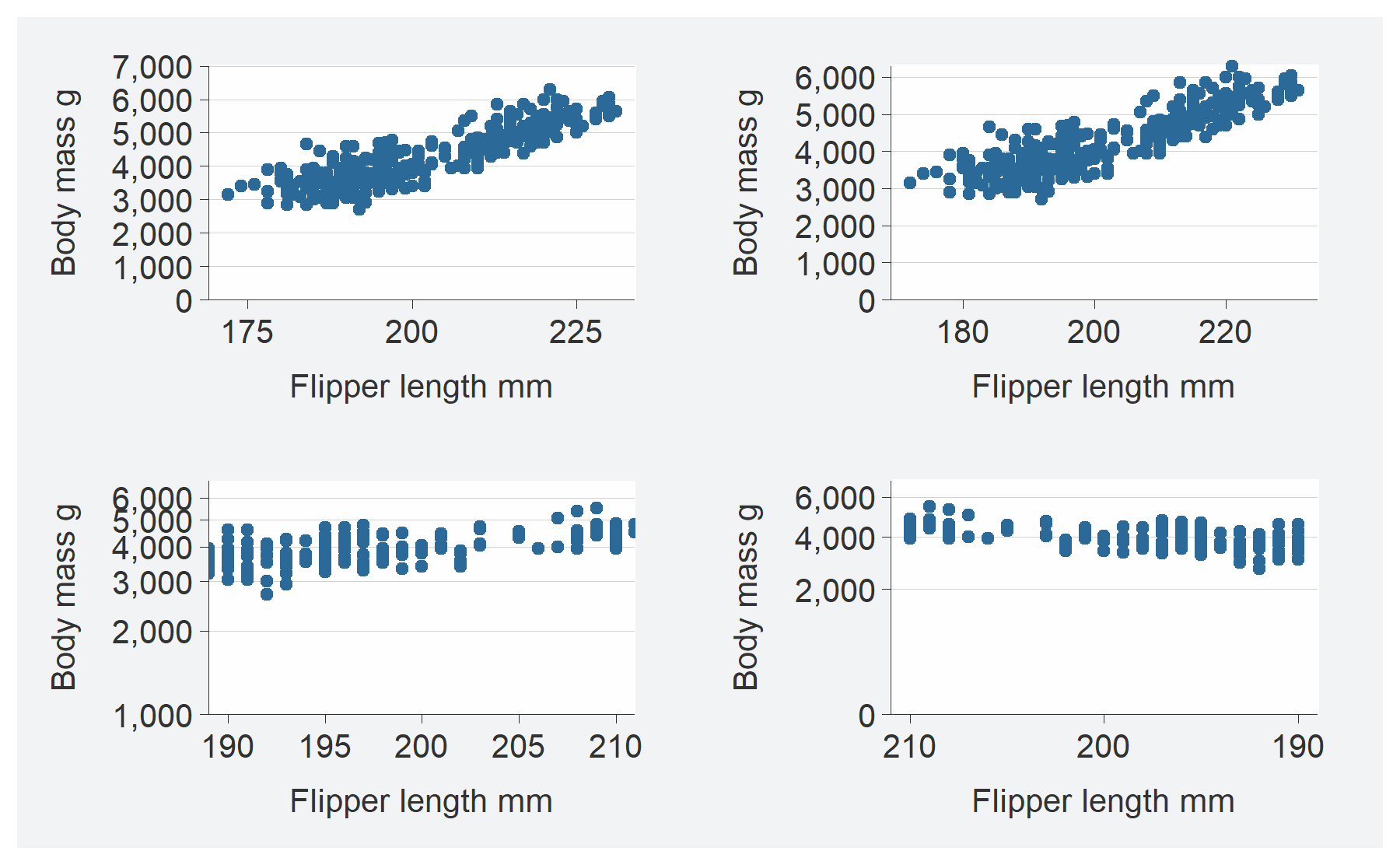### 7. Default titles converted to sentence case

Default titles are converted to sentence case with snakecase::to_sentence. All titles can be manually changed using the `*_title` arguments. However, with the default conversion, it is intended that titles are less likely to need to be manually changed than if default titles were left as variable names.

``````penguins |>
ggplot() +
geom_blank(aes(x = flipper_length_mm, y = body_mass_g)) +
labs(title = "Penguins body mass by flipper length",
subtitle = " Palmer Archipelago, Antarctica",
x = "Flipper length (mm)",
caption = "Source: Gorman, 2020")``````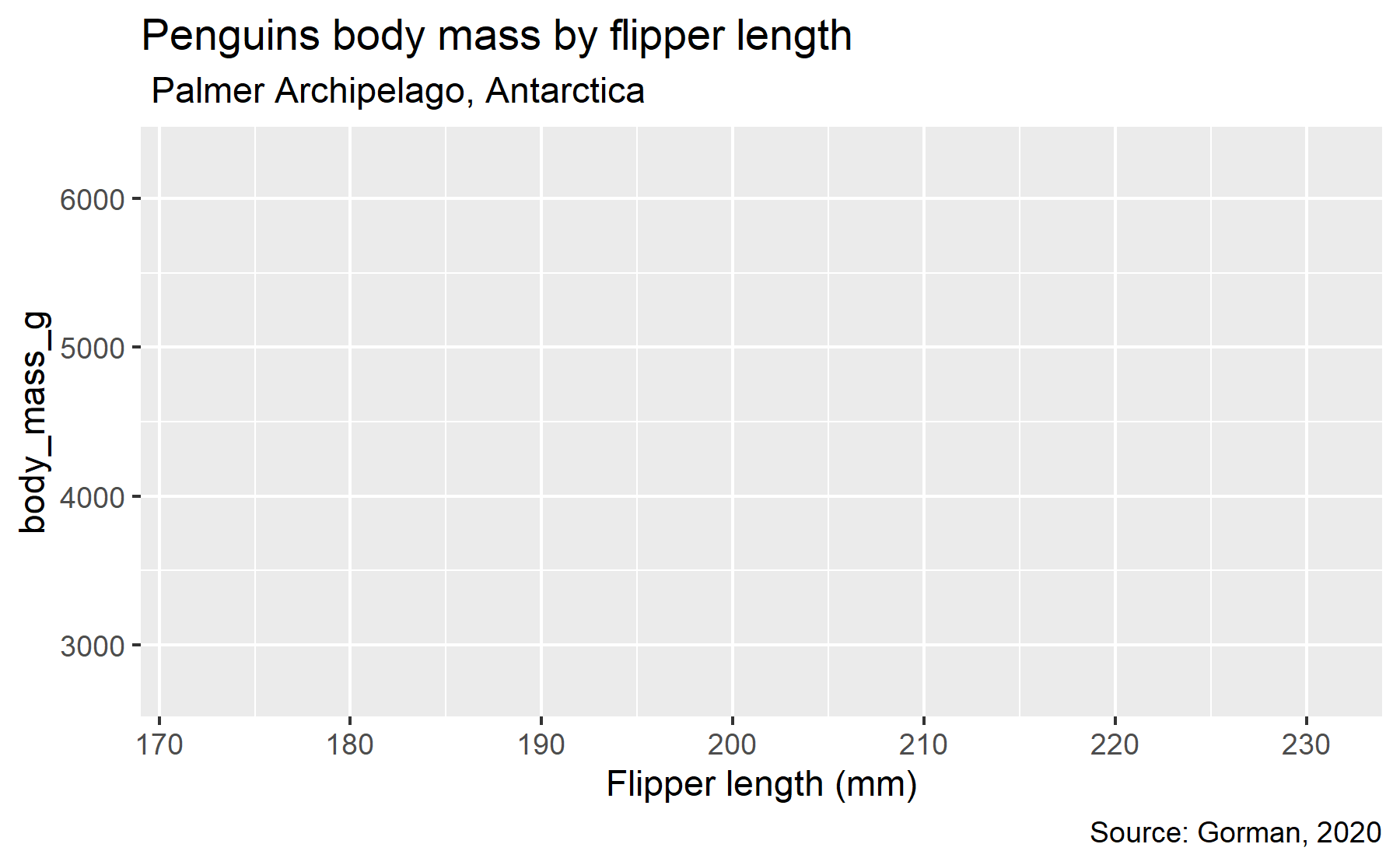``````penguins |>
gg_blank(
x = flipper_length_mm,
y = body_mass_g,
title = "Penguins body mass by flipper length",
subtitle = " Palmer Archipelago, Antarctica",
x_title = "Flipper length (mm)",
caption = "Source: Gorman, 2020")``````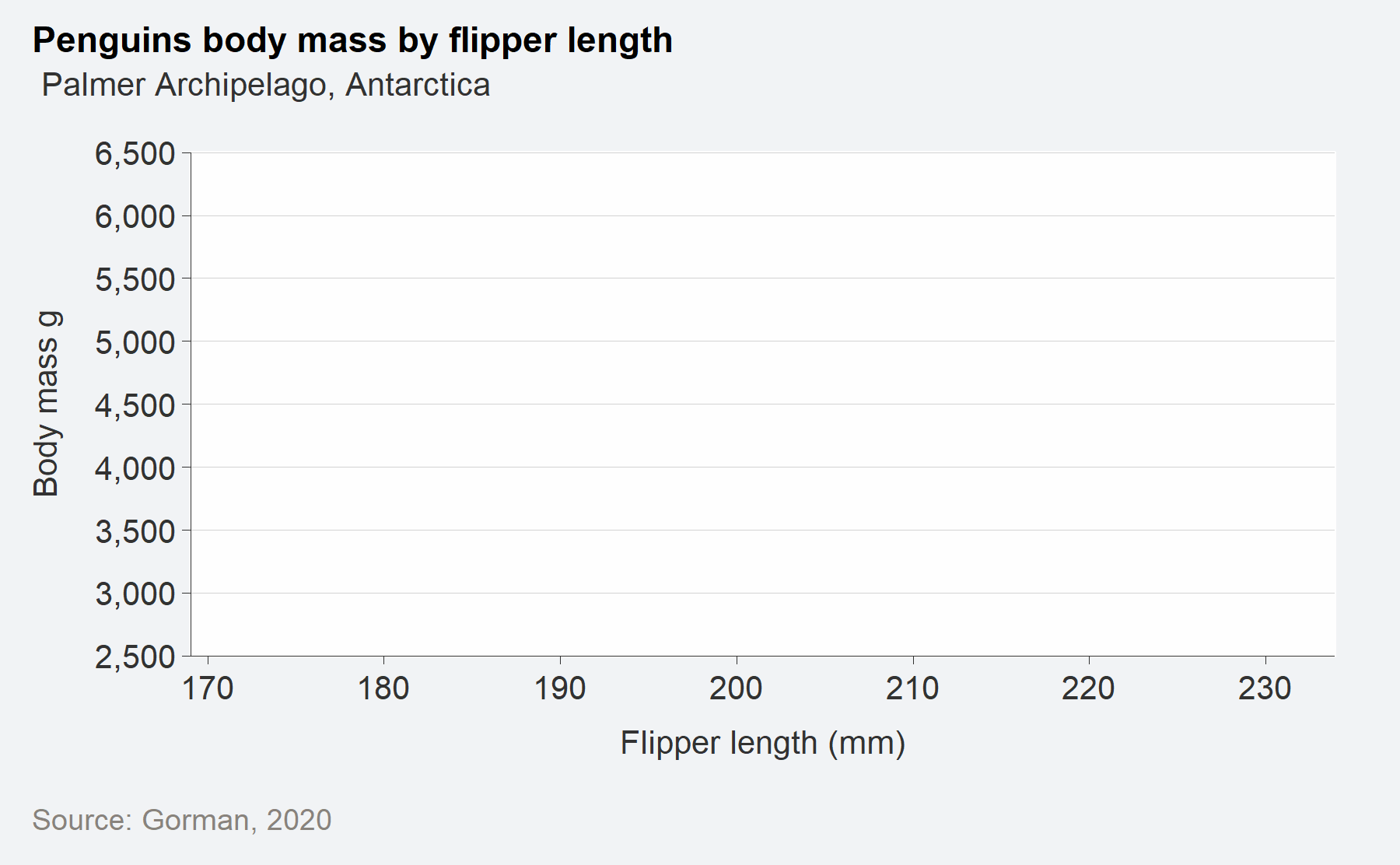### 8. A theme argument to customise the look and feel

A `theme` argument is available. This allows users to make content that has their required look and feel. By using the `theme` argument, the theme will control all theme aspects, except (1) the placement of the legend and (2) gridline removal. However, if users want their theme to adjust everything, then they can `+` their theme as a layer instead.

``````penguins |>
gg_point(x = flipper_length_mm,
y = body_mass_g,
col = sex,
facet = species,
pal = c("#2596be", "#fc7c24"),
theme = theme_grey())``````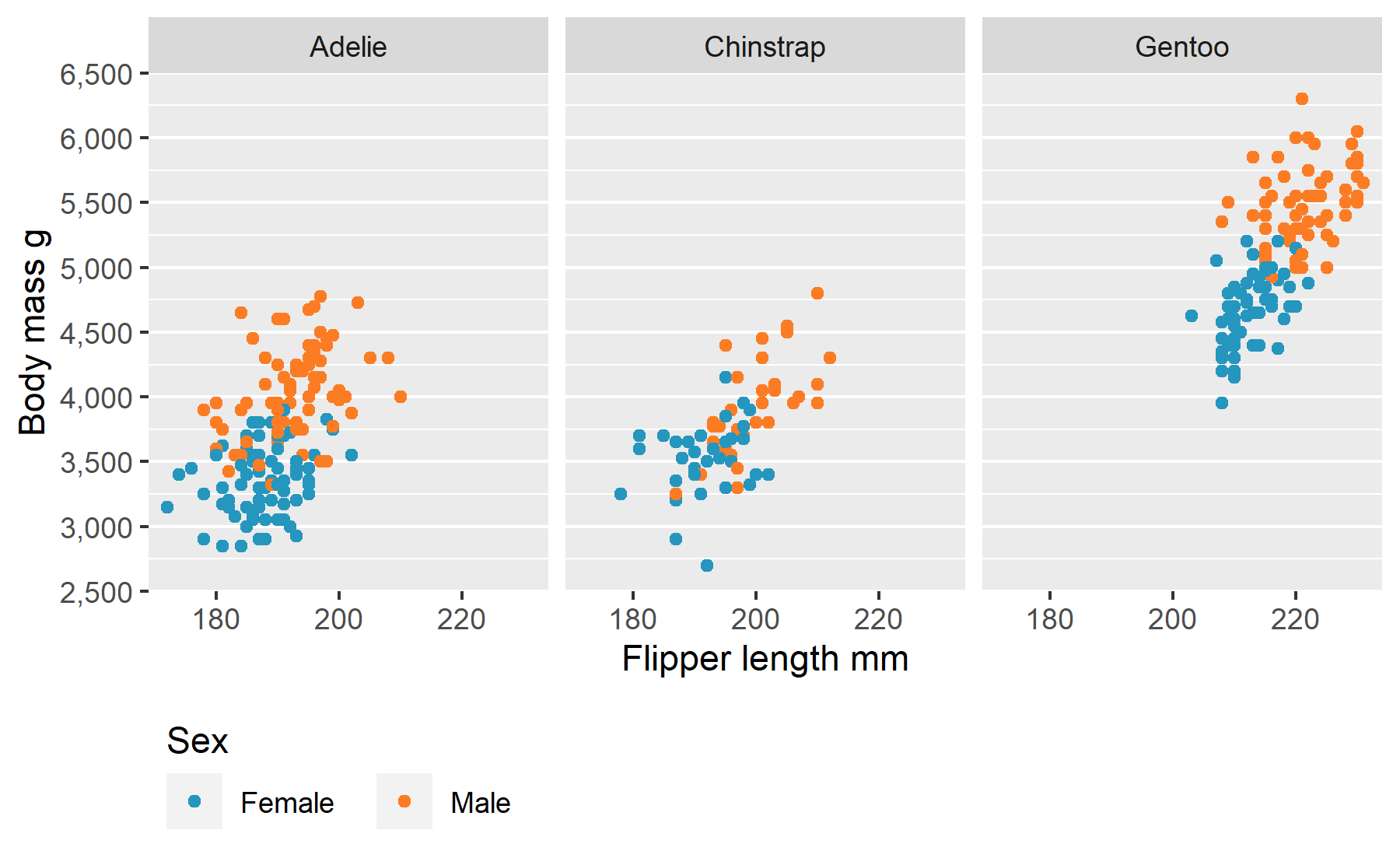``````penguins |>
gg_point(x = flipper_length_mm,
y = body_mass_g,
col = sex,
facet = species,
pal = c("#2596be", "#fc7c24")) +
theme_grey()``````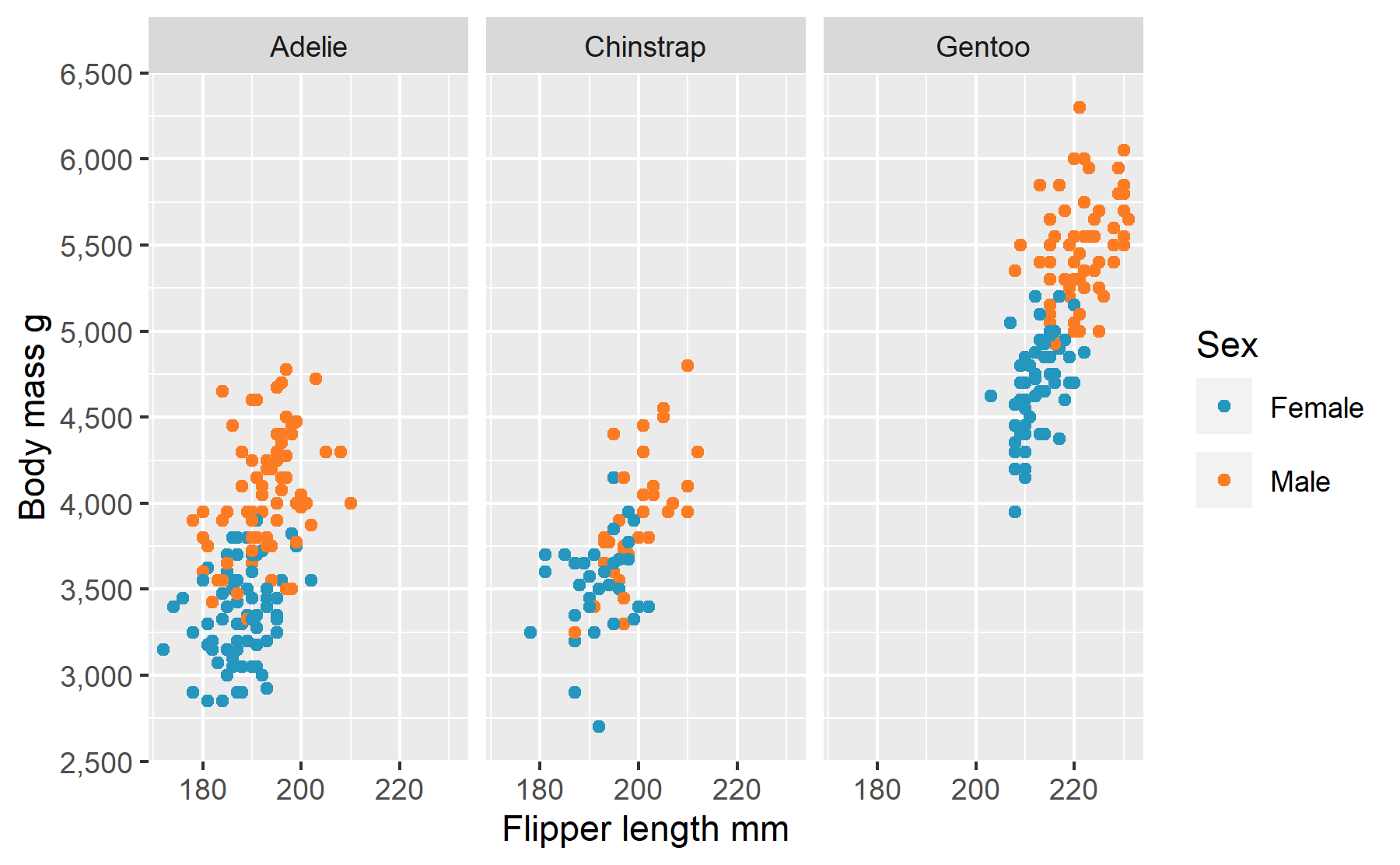### 9. A gg_theme function to create a quick theme

A `gg_theme` function is provided to allow users to quickly adjust the default ggblanket theme by changing text, background colours, axis lines, ticks and gridlines etc.

``````custom_theme <- gg_theme(
text_size = 9,
plot_background_pal = "#000000",
panel_background_pal = "#232323",
panel_grid_pal = "#000000",
text_pal = "#d3d3d3"
)

penguins |>
gg_point(
x = flipper_length_mm,
y = body_mass_g,
col = species,
theme = custom_theme) ``````